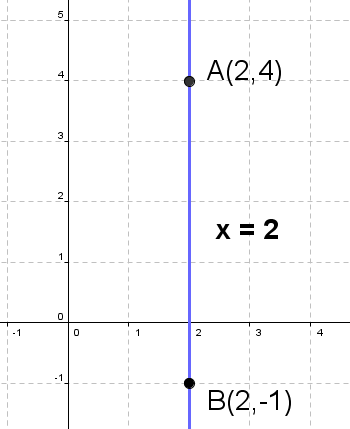Math Calculators, Lessons and Formulas

It is time to solve your math problem

mathportal.org

# Two point form calculator

This online calculator can find and plot the equation of a straight line passing through the two points. The calculator will generate a step-by-step explanation on how to obtain the result.

Line through two points
show help ↓↓ examples ↓↓
 General form (default) Slope y-intercept form
Hide steps
Find approximate solution
working...
examples
example 1:ex 1:
Determine the equation of a line passing through the points $(-2, 5)$ and $(4, -2)$.
example 2:ex 2:
Find the slope - intercept form of a straight line passing through the points $\left( \frac{7}{2}, 4 \right)$ and $\left(\frac{1}{2}, 1 \right)$.
example 3:ex 3:
If points $\left( 3, -5 \right)$ and $\left(-5, -1\right)$ are lying on a straight line, determine the slope-intercept form of the line.

## How to find equation of the line determined by two points?

To find equation of the line passing through points $A(x_A, y_A)$ and $B(x_B, y_B)$ ( $x_A \ne x_B$ ), we use formula:

$${\color{blue}{ y - y_A = \frac{y_B - y_A}{x_B-x_A}(x-x_A) }}$$

Example:

Find the equation of the line determined by $A(-2, 4)$ and $B(3, -2)$.

Solution:

In this example we have: $x_A = -2,~~ y_A = 4,~~ x_B = 3,~~ y_B = -2$. So we have:

\begin{aligned} y - y_A & = \frac{y_B - y_A}{x_B-x_A}(x-x_A) \\ y - 4 & = \frac{-2 - 4}{3 - (-2)}(x - (-2)) \\ y - 4 & = \frac{-6}{5}(x + 2) \end{aligned}Multiply both sides with $5$ to get rid of the fractions.

\begin{aligned} (y - 4)\cdot {\color{red}{ 5 }} & = \frac{-6}{5}\cdot {\color{red}{ 5 }}(x + 2)\\ 5y - 20 & = -6(x + 2)\\ 5y - 20 & = -6x - 12 \\ 5y & = -6x - 12 + 20 \\ 5y & = -6x + 8 \\ {\color{blue}{ y }} & {\color{blue}{ = -\frac{6}{5}x - \frac{8}{5} }} \end{aligned}

In special case (when $x_A = x_B$ the equation of the line is:

$${\color{blue}{ x = x_A }}$$

Example 2:Find the equation of the line determined by $A(2, 4)$ and $B(2, -1)$.

Solution:

In this example we have: $x_A = 2,~~ y_A = 4,$ $x_B = 2,~~ y_B = -1$. Since $x_A = x_B$, the equation of the line is:

$${\color{blue}{ x = 2 }}$$

You can see from picture on the right that in special case the line is parallel to y - axis.

Note: use above calculator to check the results.

Search our database of more than 200 calculators